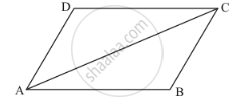Advertisement Remove all ads

# Complete the Following Statement by Means of One of Those Given in Brackets Against Each: f one pair of opposite sides are equal and parallel, then the figure is - Mathematics

MCQ
Fill in the Blanks

Complete the following statement by means of one of those given in brackets against each:

If one pair of opposite sides are equal and parallel, then the figure is ........................

#### Options

• parallelogram

• rectangle

• trapezium

Advertisement Remove all ads

#### Solution

If one pair of opposite sides are equal and parallel, then the figure is parallelogram.

Reason:In ΔABC and ΔCDA,

AB = DC Given)

AC = AC (Common)

∠BAC = ∠DCA (Because  AB || CD, Alternate interior angles are equal)

So, by SAS Congruence rule, we have

ΔABC ≅ ΔCDA

Also,

∠BCA =∠DAC (Corresponding parts of congruent triangles are equal)

But, these are alternate interior angles, which are equal.

AD || BC

Thus,  AB || CD and AD || BC

Hence, quadrilateral ABCD is parallelogram

Is there an error in this question or solution?
Advertisement Remove all ads

#### APPEARS IN

RD Sharma Mathematics for Class 9
Chapter 13 Quadrilaterals
Q 14.1 | Page 69
Advertisement Remove all ads
Advertisement Remove all ads
Share
Notifications

View all notifications

Forgot password?
Course